# c++中引用、指针、函数的深思

1. 变量（variable）的表现形式；

2. 指针的结构和原理；

3. 引用的结构和原理；

4. c语言中函数调用的本质；

5. 引用比指针的优越感；

6. 链表或者树的操作

变量在内存中的操作其实是需要经过2个步骤的：

1）找出与变量名相对应的内存地址。

2）根据找到的地址，取出该地址对应的内存空间里面的值进行操作。

int x = 5;
int *ptr = &x;

ptr即是一个指正变量名。通过指针获取这个指针指向的内存中的值称为dereference。

&：“&引用” 和“&取地址符”的区别和作用

1.定义的时候必须初始化，即& 前面有类名或类型名，&别名后面一定带 “=” （在= 左边）；
2.&后面的别名是新的名字，之前不存在。

&取地址时：

int x = 5;
int &y = x;

reference 和 pointer主要有以下3中不同点：

1）reference不需要dereference即可直接获取到指向的内存空间的值。例如上例中，直接y就可以获取reference y所指向的内存空间的值，而不需要*y来获取。

2）reference的赋值操作也不需要取地址符来赋值，可以直接通过变量名，例如上例中，int &y = x, 而不需要 int &y = &x;

3) reference 在申明的时候就必须要有初始值，而且reference变量指向的内存地址是不能变化，不像pointer那样可以很灵活的重新指向其他地址。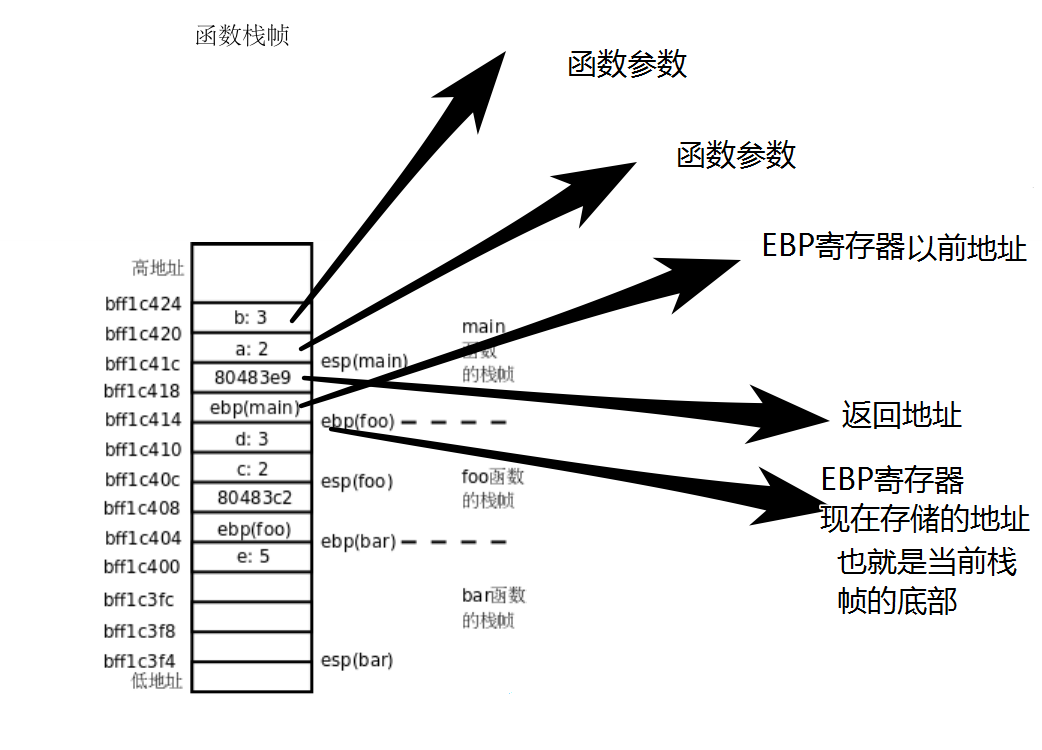#include<iostream>
int* func1(int* x);
int& func2(int &x);
int main() {
int a = 0;
std::cout << *func1(&a) << std::endl;
std::cout << &func2(a) << std::endl;
system("pause");
}
int *func1(int* x) {
(*x)++;
return x;
}
int &func2(int&x) {
x++;
return x;
}

#include<iostream>
void swap1(int* x,int* y);
void swap2(int& x,int& y);
int main() {
int x = 1;
int y = 2;
std::cout << "x=" << x << " y=" << y << std::endl;
swap1(&x, &y);
std::cout << "x=" << x << " y=" << y << std::endl;
swap2(x, y);
std::cout << "x=" << x << " y=" << y << std::endl;
}
void swap1(int*x,int*y) {
int tmp = *y;
*y = *x;
*x = tmp;
}
void swap2(int&x, int&y) {
int tmp = y;
y = x;
x = tmp;
}

#include<iostream>
int Add(const int& x, const int& y);
int main() {
int x = 1;
int y = 2;
int s = 0;
std::cout << x << " + " << y << " = " << s << std::endl; // Output : 1 + 2 = 3
}
int Add(const int& x, const int& y) {
return x + y;
}

类型名 *函数名(函数参数表列);

其中，后缀运算符括号“()”表示这是一个函数，其前缀运算符星号“*”表示此函数为指针型函数，其函数值为指针，即它带回来的值的类型为指针，当调用这个函数后，将得到一个“指向返回值为…的指针（地址），“类型名”表示函数返回的指针指向的类型”。

“(函数参数表列)”中的括号为函数调用运算符，在调用语句中，即使函数不带参数，其参数表的一对括号也不能省略。其示例如下：

int *pfun(int, int);

由于“*”的优先级低于“()”的优先级，因而pfun首先和后面的“()”结合，也就意味着，pfun是一个函数。即：

int *(pfun(int, int));

接着再和前面的“*”结合，说明这个函数的返回值是一个指针。由于前面还有一个int，也就是说，pfun是一个返回值为整型指针的函数。

我们不妨来再看一看，指针函数与函数指针有什么区别？

int (*pfun)(int, int);

通过括号强行将pfun首先与“*”结合，也就意味着，pfun是一个指针，接着与后面的“()”结合，说明该指针指向的是一个函数，然后再与前面的int结合，也就是说，该函数的返回值是int。由此可见，pfun是一个指向返回值为int的函数的指针。

虽然它们只有一个括号的差别，但是表示的意义却截然不同。函数指针的本身是一个指针，指针指向的是一个函数。指针函数的本身是一个函数，其函数的返回值是一个指针。

《用函数指针作为函数的返回值》

int (*ff(int))(int *, int);

int (*(ff(int)))(int *, int);                            // 用括号将ff(int)再括起来

接着与前面的“*”结合，说明ff函数的返回值是一个指针。然后再与后面的“()”结合，也就是说，该指针指向的是一个函数。

int (*PF)(int *, int);

typedef int (*PF)(int *, int);

PF ff(int);

#include<stdio.h>
void fun(int *s)
{
*s=100;
}
int main()
{
int a=10;
int *q;
q=&a;
printf("%d\n",*q);
fun(q);
printf("%d\n",*q);
return 0;
}

10
100

#include<stdio.h>

void  fun(int  *p){
int  b=100;
p=&b;
}

int main(){
int  a=10;
int  *q;
q=&a;
printf("%d\n",*q);
fun(q);
printf("%d\n",*q);
return  0;
}

10
10

#include<stdio.h>

void  fun(int  **p){
int  b=100;
*p=&b;
}

int main(){
int  a=10;
int  *q;
q=&a;
printf("%d\n",*q);
fun(&q);
printf("%d\n",*q);
return  0;
}

10
100

#include<stdio.h>
#include<stdlib.h>

void  myMalloc(char  *s){
s=(char*)malloc(100);
}

int main()
{
char  *p=NULL;
myMalloc(p);
if(p==NULL)
printf("P is not changed!\n");
else{
printf("P has been changed!\n");
free(p);
}
return 0;
}

P is not changed!

#include<stdio.h>
#include<stdlib.h>

void  myMalloc(char  **s){
*s=(char*)malloc(100);
}

int main()
{
char  *p=NULL;
myMalloc(&p);
if(p==NULL)
printf("P is not changed!\n");
else{
printf("P has been changed!\n");
free(p);
}
return 0;
}

P has been changed!

int a=50;

int *p=&a;// '&'的作用就是把a变量在内存中的地址给提取出来，具体后面解释。

*p=5;//这就是解引用，*解释了 p对常量 50的内存地址的引用，解释结果就是直接去寻找p所指内容，因为p指向a，所以a的内容将被修改为5，而不是50。

在指针操作中，&还有一种叫法叫做“脱去解引用”，在这个表达式中&*p（假设p是指针），&脱去了*对a的解引用，从而得到抵消的效果，得到应该是p所指的变量的地址。

## 什么时候需要传递二级指针？

void fun(int *p)，指针参数p的副本为_p，编译器使_p=p，_p和p指向相同的内存空间，如果在函数内修改了_p所指向的内容，就会导致p的内容也做相应的改变；

void fun(int **p)，如果函数参数是指针的地址，则可以通过该参数p将新分配或新指向的内存地址传递出来，这样就实现了有效的指针操作。

#include<stdio.h>
#include<stdlib.h>

char* myMalloc() {
char *s = (char*)malloc(100);
char a ="asdhjf";
s = a;
return s;
}
int main()
{
char  *p = NULL;
p = myMalloc();
if (p == NULL)
printf("P is not changed!\n");
else {
printf("P has been changed\n");
free(p);
}
return 0;
}

P has been changed!

#include <iostream>
using namespace std;
int main() {
char *p;
void *p1;
int a = 10;
p1 = &a;
//p = (char *)10;
p =(char*) malloc(sizeof(char) * 12);
system("PAUSE");
}

## 指针数组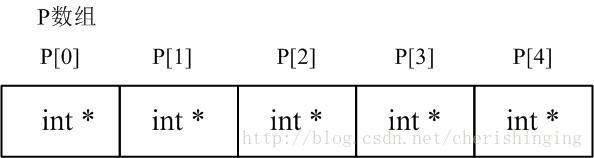## 数组指针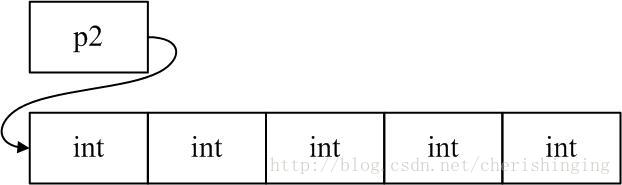## 函数指针

#include <stdio.h>

int* fun(int p1,int p2)
{
int i = 0;
i = p1 + p2;
return i;
}
int main()
{
int* (*pf)(int p1,int p2);
int *p;
pf = &fun;
p = (*pf)(1,2);
return 0;
}

## 函数指针数组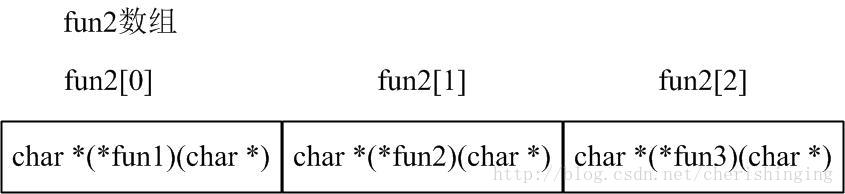#include <stdio.h>
#include <string.h>
char* fun1(char* p)
{
printf("%s\n",p);
return p;
}
char* fun2(char* p)
{
printf("%s\n",p);
return p;
}
char* fun3(char* p)
{
printf("%s\n",p);
return p;
}
int main()
{
char* (*pf)(char* p);
pf= fun1; // 可以直接用函数名
pf= &fun2; // 可以用函数名加上取地址符
pf= &fun3;
pf("fun1");
pf("fun2");
pf("fun3");
return 0;
}

## 指向函数指针数组的指针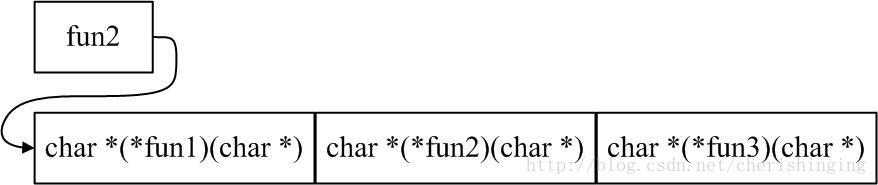#include <stdio.h>
#include <string.h>
char* fun1(char* p)
{
printf("%s\n",p);
return p;
}
char* fun2(char* p)
{
printf("%s\n",p);
return p;
}
char* fun3(char* p)
{
printf("%s\n",p);
return p;
}
int main()
{
char* (*a)(char* p);
char* (*(*pf))(char* p);
pf = &a;
a= fun1;
a= &fun2;
a= &fun3;
pf("fun1");
pf("fun2");
pf("fun3");
return 0;
}

=========================未完待续====================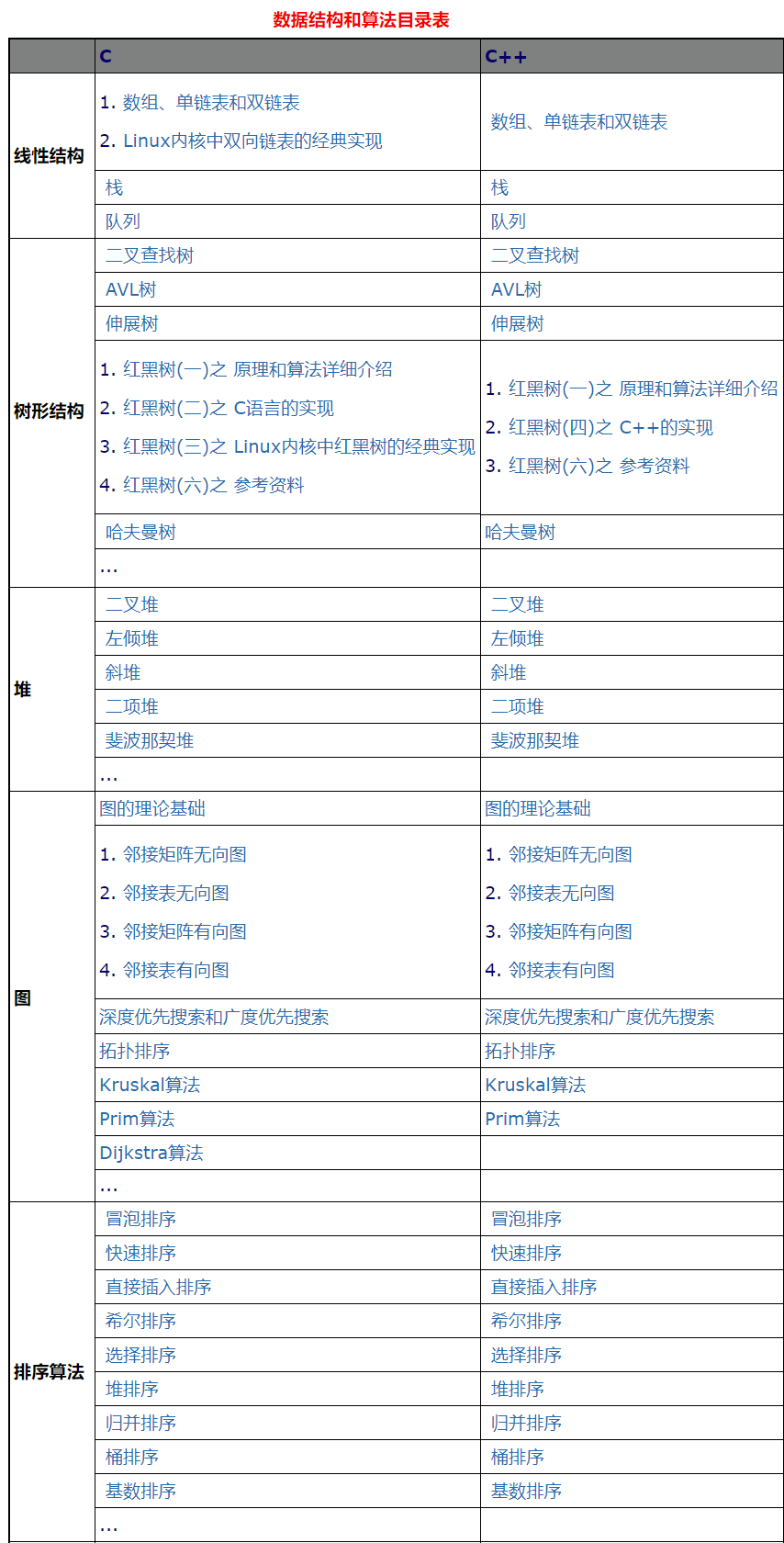http://www.cnblogs.com/VIPler/p/4319313.html

https://yq.aliyun.com/articles/297093

https://blog.csdn.net/cherishinging/article/details/72229626

https://blog.csdn.net/majianfei1023/article/details/46629065

http://www.cnblogs.com/oomusou/archive/2006/10/22/536184.html

https://www.cnblogs.com/tangxiaobo199181/p/7989464.html

https://blog.csdn.net/tianxiaolu1175/article/details/46889523.html

http://www.cnblogs.com/AndyJee/p/4630153.html©️2019 CSDN 皮肤主题: 技术黑板 设计师: CSDN官方博客